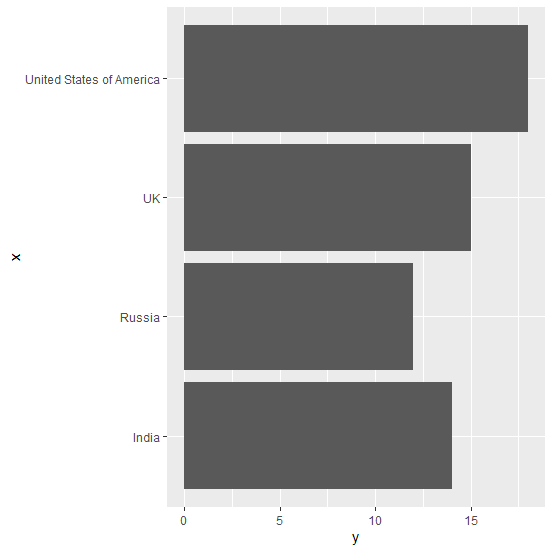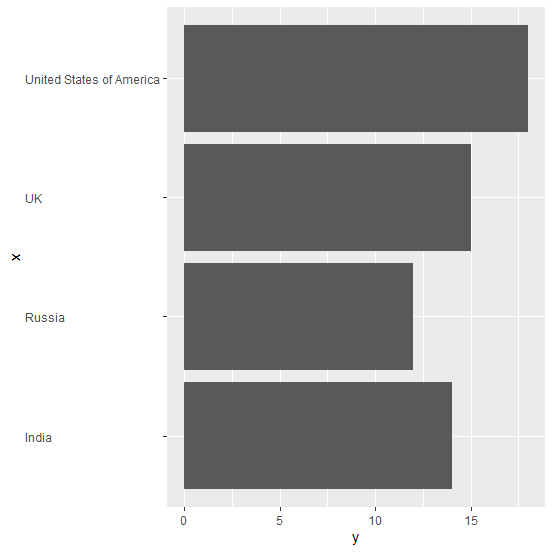# How to set the alignment of labels in horizontal bar plot to left in R?

When we create a horizontal bar plot using ggplot2 package, the labels of the categorical variable are aligned to the right-side of the axis and if the size of these labels are different then it looks a little ambiguous. Therefore, we might want to set the alignment of the labels to left-side and this can be done by using theme function of ggplot2 package.

## Example

Consider the below data frame:

> df<-data.frame(x,y)
> df

## Output

    x     y
1 India  14
2 UK     15
3 Russia 12
4 United States of America 18

## Example

> library(ggplot2)
> ggplot(df,aes(x,y))+geom_bar(stat="identity")+coord_flip()

## Output:Creating the horizontal bar plot with categorical variable labels aligned to left-side:

## Example

> ggplot(df,aes(x,y))+geom_bar(stat="identity")+coord_flip()+theme(axis.text.y=element_text(hjust=0))

## Output: